# Structure of Atom Class 9 – Science Chapter 4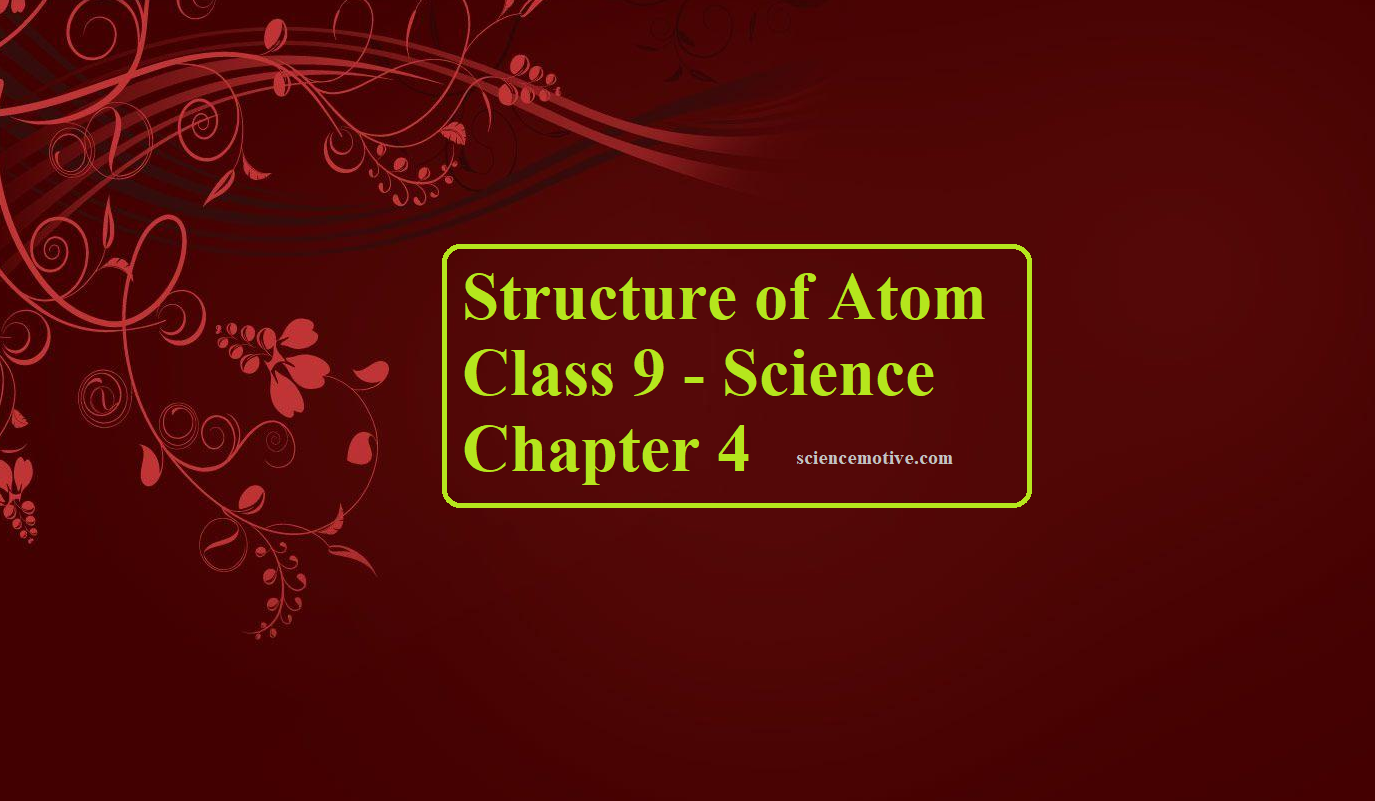# Structure of Atom Class 9 – Science Chapter 4

Structure of Atom Class 9

 Structure of Atom The term atom was introduced by Dalton and is defined as the smallest particle of an element that retains all its properties and identity during a chemical reaction.

SUB – ATOMIC PARTICLE

(i) Discovery of Electron
Electron was discovered by J J Thomson through the Cathode ray discharge tube experiment. A cathode-ray tube is made of glass containing two thin pieces of metal (called electrodes) sealed in it. The electrical discharge through the gases could be observed only at very low pressures and at very high voltages.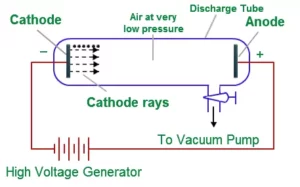When a very high voltage (about 10,000 volts) is applied between the two electrodes, no electric discharge occurs at normal pressure. When the pressure of the gas inside the tube is less than 1 mm of mercury, a dark space appears near the cathode. When the pressure is reduced to 0.01 mm Hg, it fills the whole tube. When the pressure is further reduced (10-4 mm Hg), the electric discharge passes between the electrodes and the tube begins to glow. This is due to the striking of some invisible rays from the cathode. These rays which start from the cathode and move away from it, in straight lines are called cathode rays or cathode ray particles.
These rays can be further checked by making a hole in the anode and coating the tube behind the anode with phosphorescent material like zinc sulphide. When these rays strike the zinc sulphide coating, a bright spot on the coating is developed.

Properties of Cathode Rays
1. The cathode rays start from the cathode and move towards the anode.
2. They are invisible, but their behaviour can be observed with the help of fluorescent or phosphorescent materials.
3. In the absence of an electrical or magnetic field, these rays travel in straight lines.
4. In the presence of the electric or magnetic field, the cathode rays behave similarly to that of negatively charged particles. From this, it is clear that the cathode rays consist of negatively charged particles called electrons.
5. The characteristics of cathode rays (electrons) do not depend upon the material of electrodes and the nature of the gas present in the cathode rays.
6. These rays possess kinetic energy and hence can do mechanical work.
7. They can produce x-rays when incident on metals with high atomic mass.

Charge on the Electron (e):
R.A. Millikan determined the charge on the electrons by a method known as the ‘oil drop experiment’. He found the charge on the electron to be 1.6022 × 10–19 C.

Mass of Electron (me):
The mass of the electron (me) was determined as follows:
Mass of electron (me) = e / e/me = 1.6022 × 10-19/1.758 × 1011
Mass of Electron = 9.1 × 10-31 kg

Discovery of Protons:
E. Goldstein modified the discharge tube experiment by perforated (with small holes) cathode. After evacuating the tube and applying high voltage, he found that some rays were emitting behind the cathode and moving in the opposite direction of cathode rays. These rays deflect to the negative plate of the electric field. So they carry a positive charge and were called anode rays or canal rays.

Discovery of Neutrons:
Neutrons were discovered by James Chadwick by bombarding a thin sheet of beryllium with α-particles.
4Be9 + 2He46C12 + 0n1
They are electrically neutral particles having a mass slightly greater than that of the protons.

Characteristics of Sub–Atomic Particles:

 Sub Atomic Particle Symbol Discoverer Absolute Charge (in Coulomb) Relative Charge Mass In kg Electron e J.J. Thomson -1.6022 × 10-19 -1 9.01 × 10-31 Proton p E. Goldstein +1.6022 × 10-19 +1 1.6726 × 10-27 Neutron n James Chadwick 0 0 1.675 × 10-27

This is all about various experiments involved in The Discovery of Subatomic Particles.

Atomic Number: It is the number of protons present in the nucleus or the number of electrons present outside the nucleus.
It is denoted by the symbol ‘Z’.
Atomic number (Z) = nuclear charge or number of protons (p) = number of electrons (e)

Mass Number: It is the total number of protons and neutrons in an atom. Or, it is the total number of nucleons in an atom. It is denoted by ‘A’.
i.e. Mass number (A) = no. of protons (p) + no. of neutrons (n) or, A = p + n
By knowing the atomic number and mass number, we can calculate the number of neutrons as:
n = A – Z If an element X has the atomic number Z and the mass number A, it is denoted as ZXA

Types of Atomic Species
1. Isotopes: Atoms of the same element having the same atomic number but a different mass number. For example, isotopes of Hydrogen are 11H, 12H, 13H.

2. Isobars: Atoms of different elements having the same mass number but a different atomic number, e.g., 1840Ar, 1940K, 20Ca40

3. Isotones: Atoms of different elements which contain the same number of neutrons. e.g., 614C, 715N, 816

Structure of Atom Class 9

ATOMIC MODELS: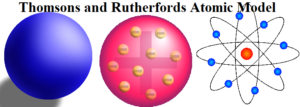Thomsons and Rutherfords Atomic Model

Thomsons and Rutherford’s Atomic Model is about the most important atomic models proposed during the study of the structure of atoms.

Thomson’s Model of Atom: J. Thomson proposed the first atomic model, which is known as the plum pudding or raisin pudding, or watermelon model. According to this model, an atom has a spherical shape in which the positive charge is uniformly distributed. The electrons are distributed in it, just like the seeds are distributed in a watermelon or plums are distributed in a pudding. An important feature of this model is that the mass of the atom is assumed to be uniformly distributed over the atom. Also, the total positive charge in an atom is equal to the total negative charge and hence the atom is electrically neutral.

Rutherford’s Nuclear Model of Atom:
Rutherford proposed an atomic model based on his α–particle scattering experiment. He bombarded a very thin gold foil (approximately 10-7m thickness) with α–particles.
Experiment: A stream of high-energy α–particles from a radioactive source was directed at a thin gold foil. The thin gold foil had a circular fluorescent zinc sulphide screen around it. Whenever α–particles struck the screen, a tiny flash of light was produced at that point.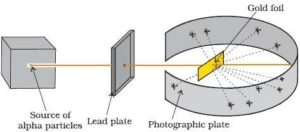Observations: The important observations made by Rutherford are:
1. Most of the α– particles passed through the gold foil without any
2. A small fraction of the α–particles were deflected by small
3. A very few α– particles (∼1 in 20,000) bounced back, that is, were deflected by nearly 180°.
Conclusions: From the above observations, Rutherford made the following conclusions:
1. Since most of the α–particles passed through the foil without any deviation, most space in the atom is empty.
2. A few positively charged α– particles were deflected. This is because the positive charge of the atom is concentrated in a very small volume at the centre called the nucleus.
3. The volume occupied by the nucleus is negligibly small as compared to the total volume of the atom. The radius of the atom is about 10–10 m, while that of the nucleus is 10–15

On the basis of the above observations and conclusions, Rutherford proposed the nuclear model (Planetary model) of an atom. According to this model:

1. All the positive charges and most of the mass of the atom is concentrated in an extremely small region called the nucleus.
2. Electrons are revolving around the nucleus at a very high speed in circular paths called an orbit.
3. Electrons and the nucleus are held together by electrostatic forces of attraction.

Drawbacks of Rutherford Model of an Atom:
Rutherford’s model cannot explain the stability of the atom.
According to Maxwell, electrons should emit electromagnetic radiation as any accelerated charged particle has the tendency of emitting electromagnetic radiation. Rutherford also stated that the electrons revolve around the nucleus in their orbits. Hence, due to the kinetic motion of the electrons, the energy of the moving electrons decreases continuously and ultimately electrons will fall inside the nucleus. Therefore, when Rutherford’s model was mixed with Maxwell’s theory, it was realized that the model is not appropriate.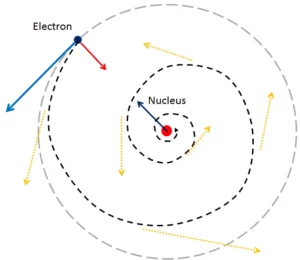Structure of Atom Class 9

Bohr’s Model of Atom: The general features of the structure of the hydrogen atom and its spectrum were first explained by Niels Bohr. The important postulates of his theory are:

1. The electron in the hydrogen atom can move around the nucleus in circular paths of fixed radius and energy. These paths are called orbits or stationary states or allowed energy states. These energy levels are numbered as 1,2,3 etc or as K, L, M, N, etc. These numbers are known as Principal quantum numbers.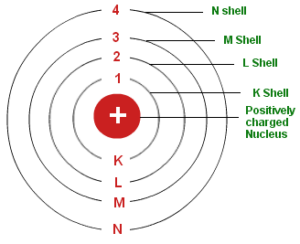2. The energy of an electron in an orbit does not change with time. However, when an electron absorbs energy, it will move away from the nucleus (i.e. to a higher energy level) and when it loses energy, it will move towards the nucleus (i.e. to a lower energy level)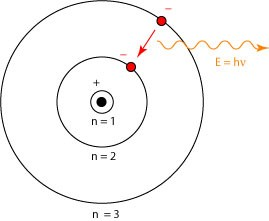3. The radius of orbits can be given by the equation: rn = a0 n2 where a0 = 52.9
Thus, the radius of the first stationary state is 52.9 pm (called the Bohr radius). As n increases, the value of r will increase.
4. The energy of the electron in an orbit is given by the expression: En = -RH (1/n2), where n = 1,2,3…… and RH is a constant called Rydberg constant. Its value is 2.18×10-18. The energy of the lowest state (the ground state) is given by E1 = –2.18×10–18J. As the value of n increases, the energy of the electron also increases.
5. The frequency of radiation absorbed or emitted when the transition occurs between two stationary states that differ in energy by ΔE, is given by: ν = ΔE/h = E2 – E1/h
Where E1 and E2 are the energies of lower and higher energy levels respectively. This expression is commonly known as Bohr’s frequency rule.
6. The angular momentum of an electron is an integral multiple of h/2π. i.e. mevr = nh/2π
Where me is the mass of the electron, v is the velocity of the electron and r is the radius of Bohr orbit. n = 1,2,3… Thus an electron can move only in those orbits whose angular momentum is an integral multiple of h/2π. So only certain fixed orbits are allowed.

Limitations of Bohr’s Atomic Model: Bohr atom model could explain the stability and line spectra of the hydrogen atom and hydrogen-like ions (e.g. He+, Li2+, Be3+ etc). But it has the following limitations:
1. It could not explain the fine spectrum of the hydrogen atom.
2. It could not explain the spectrum of atoms other than the hydrogen atom.
3. It was unable to explain the splitting of spectral lines in the presence of the electric field (Stark effect) and in the magnetic field (Zeeman effect).
4. It could not explain the ability of atoms to form molecules by chemical bonds.
5. It did not consider the wave character of matter and Heisenberg’s uncertainty principle.

Valency: An atom is stable if it has a duplet or octet configuration. A duplet or octet configuration is also known as a noble gas structure or a noble gas configuration. Atoms react in order to have a noble gas structure. The electrons present in the outermost shell of an atom are known as valence electrons. The combining capacity of the atoms of elements, that is, their tendency to react and form molecules with atoms of the same or different elements is called valency.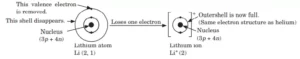Structure of Atom Class 9